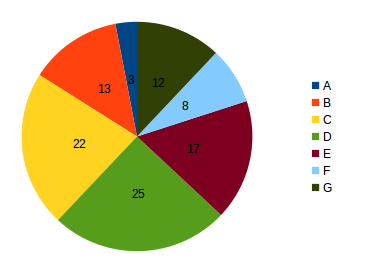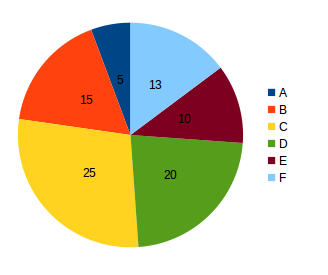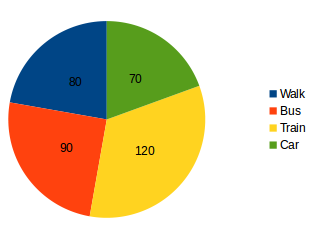## Data Interpretation For SBI PO : Set – 62

There are seven different types of tyres manufactured by a factory. The pie charts show the percentage of sales of the tyres in a city in two consecutive months of September and October. The total number of type B tyres sold in September and October were 1560 and 2250 respectively.

SeptemberOctober1) What is the maximum difference between the number of tyres of any two types sold taken together for September and that of other two types sold for October?

a) 3390

b) 5430

c) 4523

d) 4230

e) None of these

b) 5430

To find the maximum difference let us take the minimum values from September and the maximum values from October.

From September = 960+360 = 1320.

From October = 3750 +3000 = 6750.

The difference = 6750-1320 = 5430.

2)  For how many types of tyres, there is an absolute decrease in the number sold in October over September?

a) 0

b) 1

c) 2

d) 3

e) None of these

b)

There is only one  decrease is E.

3) The total sales of which of the following pairs of types of tyres in September was approximately equal to F type of tyres in October?

a) A & C

b) C & E

c) B & D

d) A & B

e) None of these

d) A & B

Sale of F type tyres in October = 1950.

In September

Sale of A and C = 360 + 2640 = 3000

Sale of C and E= 2640 + 2040 = 4680

Sale of B and D= 1560 + 3000 = 4560

Sale of A and B=360 + 1560 = 1920

4) What is the difference in total sales between September and October in respect to only those types where there was an increase in sale?

a) 6520

b) 3540

c) 4530

d) Can’t be determined

e) None of these

b)

For E, there is a decrease in sales and for D, the sales for the two years are equal.

The total sale of all tyres except D and E in September = 12000 – 3000 – 2040 = 6960

The total sale of all tyres except D and E in October = 15000 – 3000 – 1500 = 10500

The required difference = 10500 – 6960 = 3540.

5) The number of tyres of type D and E sold in September is approximately what percent of the number of tyres sold for these types in October?

a) 85%

b) 67%

c) 145%

d) 112%

e) None of these

d)

Number of tyres of type D and E sold in September = 3000 + 2040 = 5040

Number of tyres of type D and E sold in October = 3000 + 1500 = 4500

Hence the required percentage = {(5040/4500)} x 100 = 112%

D.6-10) The pie-chart given below represents the number of students using different transport to a school in which total number of students is 2160.
Answer the questions based on the following diagram.

Values are given in angle6. The number of students who come to school by car is

a) 70

b) 290

c) 420

d) 480

e) None of these

c)

The Central angle of car = 360-(80+90+120)=70º

total Students 2160

Students who come by car = (2160/360)*70=420

7. The ratio of the number of students who come to school by car to the number of students who come to school by bus is

a) 21: 24

b) 21: 27

c) 36: 27

d) 36: 21

e) None of these

b)

The central angle of car = 360 -(80+90+120)=70º

Central angle of bus is 90º

the required ratio is 70:90 or 21:27

8) The total number of students coming to school either by walking or by bus is

a) 480

b) 540

c) 1020

d) 170

e) None of these

c)

The total no.of students is 2160

The Sum of Central angles of students who come by bus or walking = 80º+90º=170º

The required ratio is (2160/360)*170=1020

9) The number of students who don’t come to School by train is

a) 720

b) 1020

c) 2040

d) 1440

e) None of these

d)

The no of students who come to school by train = (216/360)*120=720

The number of students who do not come to school by train = 2160-720=1440

10) The number of students coming to school by bus exceeds the number of students coming to school walking by

a) 10%

b) 12.5%

c) 11%

d) 11.5%

e) None of These